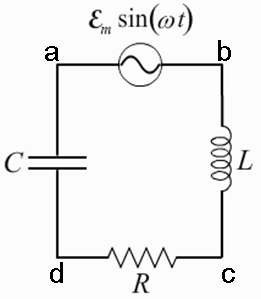# Problem: A circuit is constructed with an AC generator, a resistor, capacitor and inductor as shown. The generator voltage varies in time as ε =Va - Vb = εmsinωt, where εm = 120 V and ω = 737 radians/second. The values for the remaining circuit components are: R = 83 Ω, L = 112.5 mH, and C = 11.6μF.What is Z, the impedance of the circuit?

###### FREE Expert Solution

Impedance,

$\overline{){\mathbf{Z}}{\mathbf{=}}\sqrt{{\mathbf{R}}^{\mathbf{2}}\mathbf{+}{\mathbf{\left(}\mathbf{X}}^{}}}$L-XC)2

94% (243 ratings)###### Problem Details

A circuit is constructed with an AC generator, a resistor, capacitor and inductor as shown. The generator voltage varies in time as ε =Va - Vb = εmsinωt, where εm = 120 V and ω = 737 radians/second. The values for the remaining circuit components are: R = 83 Ω, L = 112.5 mH, and C = 11.6μF.What is Z, the impedance of the circuit?